## ICSE Physics Previous Year Question Paper 2017 Solved for Class 10

General Instructions :

• Answers to this Paper must be written on the paper provided separately.
• You will not be allowed to write during the first 15 minutes.
• This time is to be spent in reading the Question Paper.
• The time given at the head of this Paper is the time allowed for writing the answers.
• Section I is compulsory. Attempt any four questions from Section II.
• The intended marks for questions or parts of questions are given in brackets [ ].

Section -1 [40 Marks]
(Attempt all questions from this Section)

Question 1.
(a) A brass ball is hanging from a stiff cotton thread. Draw a neat labelled diagram showing the forces acting on the brass ball and the cotton thread. 
(b) The distance between two bodies is doubled. How is the magnitude of gravitational force between them affected ? 
(c) Why is a jack screw provided with along arm? 
(d) If the power of a motor be 100 kW, at what speed can it raise a load of50,000 N? 
(e) Which class of lever will always have MA > 1 and why? 
(a) The diagram is as shown.(b) It becomes one-fourth. This is because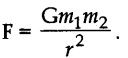(c) As a large mechanical advantage is required by the jack screw used for cutting metal, hence its handle should be long and the blades short.
(MA = effort arm / load arm)

(d) P = Fυ, therefore, υ = P/F = 100000 / 50000 = 2 ms-1

(e) Class II lever, because the effort arm is always greater than the load arm.

Question 2.
(a) Define heat capacity and state its SI unit. 
(b) Why is the base of a cooking pan generally made thick ? 
(c) A solid of mass 50 g at 150°C is placed in 100 g of water at 11°C, when the final temperature recorded is 20°C. Find the specific heat capacity of the solidfSpecific heat capacity of water – 4.2 J g -1 °C -1 ). 
(d) How is the refractive index of a material related to :
(i) real and apparent depth ?
(ii) velocity of light in vacuum or air and the velocity of light in a given medium ?
(e) State the conditions required for total internal reflection of light to take place. 
(a) It is defined as the amount of heat required in raising the temperature of a unit mass of a substance through 1°C. Its SI unit is J kg-1 K-1.

(b) It is because thicker pans can have better heat distribution and heat retention due to thermal conductivity and thermal mass.

(c) Given mass of solid ms = 50 x 10-3 kg
Mass of water mw = 100 x 10-3 kg
Temperature of solid = 1500°C
Temperature of water = 110°C
Final temperature = 200°C
Specific heat of water = 4.2 J kg-1 °C-1
Now, heat lost by the solid = heat gained by water
50 × 10-3 × c × (1500 – 200) = 100 x 10-3 × 4200 × (200 – 110)
Or  c = 581 J kg-1 °C-1

(d) (i) μ = $$\frac { real depth }{ apparent depth }$$
(ii) μ = $$\frac { c }{ \upsilon }$$

(e) (i) The incident ray should travel from the denser to the rarer medium.
(ii) The angle of incidence in the denser medium should be greater than the critical angle for the given pair of media.

Question 3.
(a) Draw a ray diagram to show the refraction of a monochromatic ray through a prism when it suffers minimum deviation. 
(b) The human ear can detect continuous sounds in the frequency range from 20 Hz to 20000 Hz. Assuming that the speed of sound in air is 330 ms-1 for all frequencies, calculate the wavelengths corresponding to the given extreme frequencies of the audible range. 
(c) An enemy plane is at a distance of 300 km from a radar. In how much time the radar will be able to detect the plane? Take velocity of radio waves as 3 × 108 m s-1. 
(d) How is the frequency of a stretched string related to : 
(i) its length?
(ii) its tension?
(e) Define specific resistance and state its SI unit. 
(a) The ray diagram is as shown: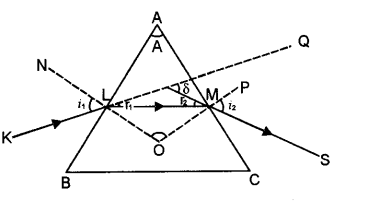(b) The corresponding wavelengths are λlowest =$$\frac { V }{ \upsilon }$$ = $$\frac { 330 }{ 20000 }$$ = 0.0165 m and λhighest = $$\frac { V }{ \upsilon }$$ =$$\frac { 330 }{ 20 }$$ = 16.5 m.

(c) Given S = 300Km = 300000 m ,c = 3 × 108ms-1, t = ?
Using $$\frac { 2S }{ \upsilon }$$ = $$\frac{2 \times 300000}{3 \times 10^{8}}$$ = 2 × 10-3 s

(d) (i) Inversely proportional to its length and
(ii) Directly proportional to the square root of tension.

(e) It is the resistance of unit cube of the material. Its SI unit is ohm-m.

Question 4.
(a) An electric bulb of resistance 500 Ω, draws a current of 0.4 A. Calculate the power of the bulb and the potential difference at its end. 
(b) State two causes of energy loss in a transformer. 
(c) State two characteristics of a good thermion emitter. 
(d) State two factors upon which the rate of emission of thermions depends. 
(e) When does the nucleus of an atom tend to be radioactive? 
(a) Given R = 500 W, I = 0.4 A, V = ?, Using V = IR, we have V = 0.4 × 500 = 200 V

(b) Copper loss and iron loss.

(c) (i) Should have high melting point and
(ii) Should have low value of work function.

(d) (i) The nature of the metal and
(ii) Temperature of the metal surface.

(e) The nucleus tends to be radioactive when it becomes unstable.

Section – II [40 Marks]
(Attempt any four questions from this Section)

Question 5.
(a) A uniform half metre rule balances horizontally on a knife edge at 29 cm mark when a weight of 20 gf is suspended from one end.
(i) Draw a diagram of the arrangement
(ii) What is the weight of the half metre rule ? 
(b) A boy uses a single fixed pulley to lift a load of 50 kgf to some height. Another boy uses a single movable pulley to lift the same load to the same height. Compare the effort applied by them. Give a reason to support your answer.
(ii) How does uniform circular motion differ from uniform linear motion ?
(iii) Name the process used for producing electricity using nuclear energy. 
(c) A pulley system with VR = 4 is used to lift a load of 175 kgf through a vertical height of 15 m. The effort required is 50 kgf in the downward direction, (g = 10 N kg-1). 
Calculate:
(i) Distance moved by the effort.
(ii) Work done by the effort.
(iii) M.A. of the pulley system.
(iv) Efficiency of the pulley system.
(a) Let the weight of the half-meter scale be W.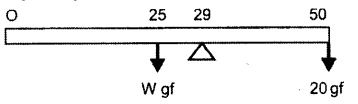To balance the half-metre scale at 29 cm mark, the 20 gf weight should be suspended from the 50 cm end as shown.
Moments due to W in anticlockwise direction = W gf × 4 cm
Moments due to 20 gf in clockwise direction = 20 gf × 21 cm
By the principle of moments we have Wgf × 4 = 20 gf × 21 20 × 21
Or W =$$\frac{20 \times 21}{4}$$ = 105 gf

(b) (i) A single fixed pulley simply changes the direction of the action of the force. Therefore, for this pulley E = L = T = 50 kgf. Thus, it has a mechanical advantage 1.
For a single movable pulley, we have L = T + T= 2T and E = T = L/2 = 50/2 = 25 kgf, therefore, its mechanical advantage is 2.
(ii) In uniform circular motion, velocity is not constant, whereas in uniform linear motion velocity is constant.
(iii) Nuclear fission

(c) Given VR = 4, L = 175 kgf, distance moved by load = dL = 15 m, E = 50 kgf, dE = ?, WE = ?, MA = ?, h = ?
distance moved by effort dE
Using the formula VR = $$\frac { distance moved by effort }{ distance moved by load }$$ = $$\frac{d_{\mathrm{E}}}{d_{\mathrm{L}}}$$ therefore, we have
dE = VR × dL 4 = 4 × 15 = 60 m
WE = Effort x dE = 50 × 10 × 60 = 30000 J
Now, MA = $$\frac { Load }{ effort }$$ = $$\frac { 175 }{ 50 }$$ = 3.5
Now, η =  $$\frac { MA }{ VR }$$ = $$\frac { 3.5 }{ 4 }$$ = 0.875 = 87.5%

Question 6.
(a) (i) How is the transference of heat energy by radiation prevented in a calorimeter ? 
(ii) You have a choice of three metals A, B and C, of specific heat capacities 900 J kg-1 °C-1, 380 / kg-1 °C-1 and 460 J kg-1 °C-1 respectively, to make a calorimeter. Which material will you select ? Justify your answer.
(b) Calculate the mass of ice needed to cool 150 g of water contained in a calorimeter of mass 50 g at 32°C such that the final temperature is 5°C.
Specific heat capacity of calorimeter 0.4 J g-1 °C-1
Specific heat capacity of water = 4.2 J g-1 °C-1
Latent heat capacity of ice 330 J g-1 
(c) (i) Name the radiations which are absorbed by greenhouse gases in the earth’s atmosphere.
(ii) A radiation X is focused by a particular device on the bulb of a thermometer and mercury in the thermometer shows a rapid increase. Name the radiation X.
(iii) Name two factors on which the heat energy liberated by a body depends. 
(a) (i) This is done by polishing the inner and outer surface of the copper
calorimeter and the space between the copper vessel and the insulating container is filled with some poor conductor like wood wool or glass wool.
(ii) A, as it will gain less energy in a given time.

(b) Given mw = 150 g, mi = ?, mC = 50 g, Ti = 0 °C, Tw = 32 °C, Tf= 5 °C
Specific heat capacity of calorimeter = 0.4 J g-1 °C-1
Specific heat capacity of water = 4.2 J g-1 °C-1
Latent heat capacity of ice 330 J gm-1
Heat lost by 150 g water + 50 g of calorimeter in falling form 32 °C to 5 °C
= 150 × 4.2 × 27 + 50 × 0.4 × 27 = 17550 J
Heat needed to melt ice = mi L = mi × 330 = 330mi
So 330mi = 17550 or mi = 17550/330 = 53.2 g

(c) (i) Infrared
(ii) Infrared
(iii) Temperature and surface area.

Question 7.
(a) A lens forms an upright and diminished image of an object when the object is placed at the focal point of the given lens.
(i) Name the lens.
(ii) Draw a ray diagram to show the image formation.
(b) A ray of light travels from water to air as shown in the diagram given below :
(i) Copy the diagram and complete the path of the ray. Given the critical angle for water is 48°.
(ii) State the condition so that total internal reflection occurs in the above diagram.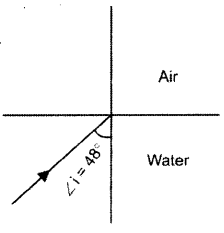(c) The diagram below shows a point source P inside a water container. Four rays A, B, C, D starting from the source P are shown upto the water surface.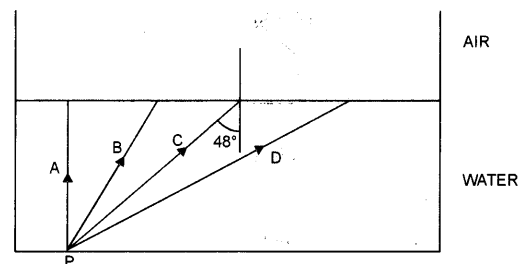(i) Show in the diagram the path of these rays after striking the water surface. The critical angle for water air surface is 48°.
(ii) Name the phenomenon which the rays B and D exhibit:
(a) Concave lens. The diagram is as shown.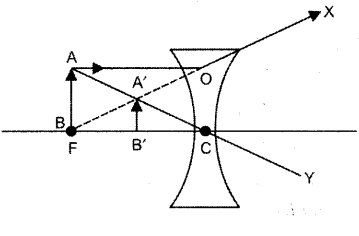(b) (i) The redrawn diagram is as shown :(ii) The angle of incidence should be greater than the critical angle.

(c) (i) The diagram is as shown.(ii) Ray B – Refraction, Ray D – Total internal reflection.

Question 8.
(a) Name the factor that determines :
(i) Loudness of the sound heard.
(ii) Quality of the note.
(iii) Pitch of the note. 
(b) (i) What are damped vibrations ?
(ii) Give one example of damped vibrations.
(iii) Name the phenomenon that causes a loud sound when the stem of a vibrating tuning fork is kept pressed on the surface of a table. 
(c) (i) A wire of length 80 cm has a frequency of 256 Hz. Calculate the length of a similar wire under similar tension, which will have frequency 1024 Hz.
(ii) A certain sound has a frequency of 256 hertz and a wavelength of 1.3 m.
(1) Calculate the speed with which this sound travels.
(2) What difference would be felt by a listener between the above sound and another sound travelling at the same speed, but of wavelength 2.6 m ? 
(a) (i) Intensity/Amplitude.
(ii) Frequency of source and relative motion between the source of sound and the listener.
(iii) The number and the frequency of the overtones present in a given sound.

(b) (i) Those vibrations which die out with time.
(ii) Vibrations of a simple pendulum.
(iii) Resonance

(c) (i) Given L1 = 80 cm, v1 = 256 Hz, L2 = ?, V2 = 1024 Hz
We know that for a vibrating string Lv = constant. Therefore, we have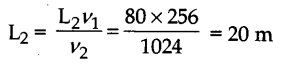(ii) (1) v = 256 Hz, λ = 1.3 m, V = ?
Using V = vλ, we have V = 256 x 1.3 = 332.8 m s-1
(2) He will hear a dull sound as the frequency of the sound wave of wavelength 2.6 m will be half the frequency (256/2 = 128 Hz) of the sound of wavelength 1.3 m.

Question 9.
(a) (i) Name the colour code of the wire which is connected to the metallic body of an appliance.
(ii) Draw the diagram of a dual control switch when the appliance is switched ‘ON’. 
(b) (i) Which particles are responsible for current in conductors ?
(ii) To which wire of a cable in a power circuit should the metal case of a geyser be connected ?
(iii) To which wire should the fuse be connected ? 
(c) (i) Explain the meaning of the statement ‘current rating of a fuse is 5A’.
(ii) In the transmission of power the voltage of power generated at the generating stations is stepped up from 11 kV to 132 kV before it is transmitted. Why ? 
(a) (i) Green and Yellow strips
(ii) The diagram is a shown.(b) (i) Electrons.
(ii) Earth wire.
(iii) Live wire.

(c) (i) It means that it can withstand a maximum current of 5 A before it “blows”.
(ii) Due to this the current flowing in the circuit decreases. As a result heat losses I2R decreases.

Question 10.
(a) Answer the following questions based on a hot cathode ray tube :
(i) Name the charged particles.
(ii) State the approximate voltage used to heat the filament.
(iii) What will happen to the beam when it passes through the electric field ? 
(b) State three factors on which the rate of emission of electrons from a metal surface depends. 
(c) (z) What are free electrons ?
(ii) Why do they not leave the metal surface on their own ?
(iii) How can they be made to leave the metal surface ? (State any two ways)